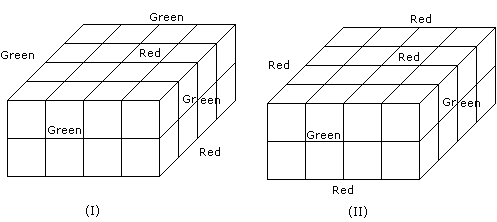# Verbal Reasoning - Cube and Cuboid

Exercise : Cube and Cuboid - Cube and Cuboid 9
Directions to Solve
A cube is cut in two equal parts along a plane parallel to one of its faces. One piece is then coloured red on the two larger faces and green on the remaining, while the other is coloured green on two smaller adjacent faces and red on the remaining. Each is then cut into 32 cubes of same size and mixed up.

1.
How many cubes have only one coloured face each ?
32
8
16
0
Explanation:8 from (I) and 8 from (II)

Therefore 8 from each.

2.
What is the number of cubes with at least one green face each ?
36
32
38
48
Explanation:24 from (I) and 14 from (II)

3.
How many cubes have two red and one green face on each ?
0
8
16
4
Explanation:None from (I) and 4 from (II)

4.
How many cubes have no coloured face at all ?
32
8
16
None
Explanation:There is no such cube in all, where all the faces are unclosured.

5.
How many cubes have each one red and another green ?
0
8
16
24